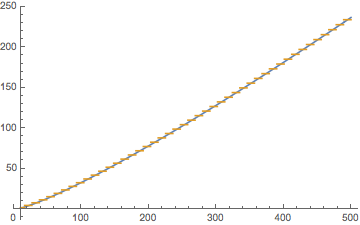# An asymptotic formula for this sum

Let $X$ be a positive real number. Can someone help me by providing an asymptotic formula for this sum.

$$\sum_{n \leq X, \; n\, \equiv\, a \mod{b}} \log{n},$$ where $a$ and $b$ are two coprime integers. Thanks in advance.

The sum $$F(X)=\sum_{n \leq X, \; n\, \equiv\, a \mod{b}} \log{n}=\sum_{p={\rm Int}\,[-a/b]}^{{\rm Int}\,[(x-a)/b]}\log(a+pb)$$ can be approximated in the large-$X$ limit by $$F_\infty(X)=\sum_{p=1}^{(X-a)/b}\log(pb)=\frac{X-a}{b}\log b+\log\Gamma\left(\frac{X-a}{b}+1\right)$$
Here is a plot of $F(X)$ (gold) and $F_\infty(X)$ (blue) for $a=5$, $b=11$.